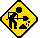## National Semiconductor 6025 Financier PR

Datasheet legend
Ab/c: Fractions calculation
AC: Alternating current
BaseN: Number base calculations
Card: Magnetic card storage
Cmem: Continuous memory
Cond: Conditional execution
Const: Scientific constants
Cplx: Complex number arithmetic
DC: Direct current
Eqlib: Equation library
Exp: Exponential/logarithmic functions
Fin: Financial functions
Grph: Graphing capability
Hyp: Hyperbolic functions
Intg: Numerical integration
Jump: Unconditional jump (GOTO)
Lbl: Program labels
LCD: Liquid Crystal Display
LED: Light-Emitting Diode
Li-ion: Lithium-ion rechargeable battery
Lreg: Linear regression (2-variable statistics)
mA: Milliamperes of current
Mtrx: Matrix support
NiMH: Nickel-metal-hydrite rechargeable battery
Prnt: Printer
RTC: Real-time clock
Sdev: Standard deviation (1-variable statistics)
Solv: Equation solver
Subr: Subroutine call capability
Symb: Symbolic computing
Tape: Magnetic tape storage
Trig: Trigonometric functions
Units: Unit conversions
VAC: Volts AC
VDC: Volts DC
 Years of production: Display type: Numeric display New price: Display color: Red Display technology: Light-emitting diode Size: 6"×3"×1" Display size: 8 digits Weight: 6 oz Entry method: Calculator arithmetic Batteries: 3×"AA" NiCd Advanced functions: Fin External power: NS adapter Memory functions: I/O: Programming model: Keystroke entry Precision: 8 digits Program functions: Memories: 1 numbers Program display: Program memory: 102 program steps Program editing: Step delete capability Chipset: Forensic result:Under construction

Stirling's formula (uncorrected):

```EX
MR/MC
MR/MC
EX
M+
/
2.7182818
X
MR
PWR
EX
MR/MC
MR/MC
EX
M+
EX
X
2
X
3.1415927
/
2
PWR
X
MR
=```